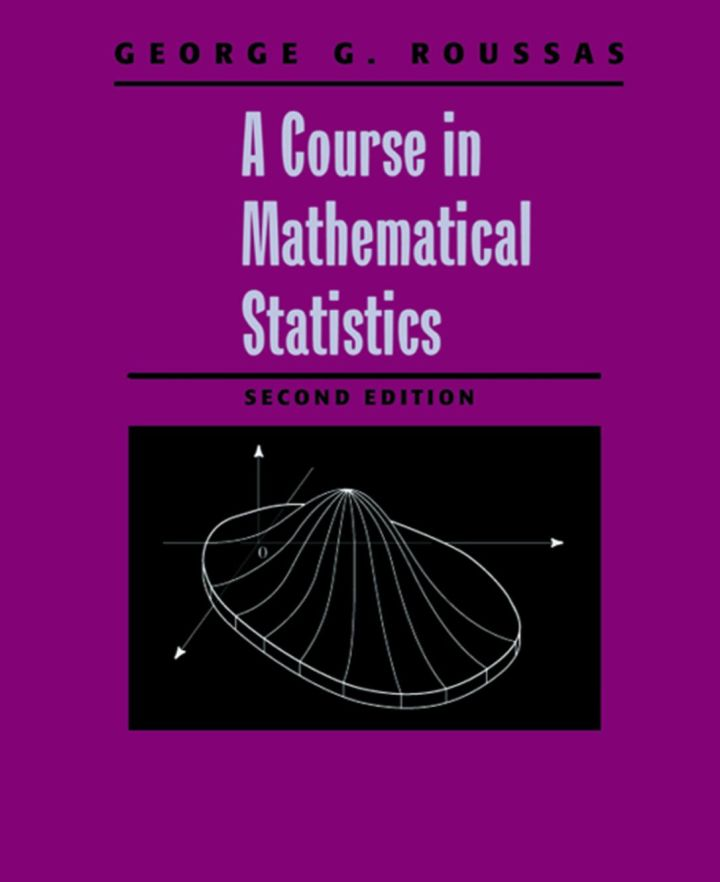# A Course in Mathematical Statistics

## A Course in Mathematical Statistics

By Roussas, George G.

### Rent or Buy eTextbook

Expires on Apr 17th, 2022
\$102

Publisher List Price: \$119.99
Savings: \$17.99

A Course in Mathematical Statistics, Second Edition, contains enough material for a year-long course in probability and statistics for advanced undergraduate or first-year graduate students, or it can be used independently for a one-semester (or even one-quarter) course in probability alone. It bridges the gap between high and intermediate level texts so students without a sophisticated mathematical background can assimilate a fairly broad spectrum of the theorems and results from mathematical statistics. The coverage is extensive, and consists of probability and distribution theory, and statistical inference.

* Contains 25% new material
* Includes the most complete coverage of sufficiency
* Transformation of Random Vectors
* Sufficiency / Completeness / Exponential Families
* Order Statistics
* Elements of Nonparametric Density Estimation
* Analysis of Variance (ANOVA)
* Regression Analysis
* Linear Models

Subject: Mathematics & Statistics -> Calculus -> Calculus2nd edition
Publisher: Elsevier S & T 03/1997
Imprint: Academic Press
Language: English
Length: 572 pages

ISBN 10: 0125993153
ISBN 13: 9780125993159
Print ISBN: 9780125993159

Live Chats